# （数据科学学习手札43）Plotly基础内容介绍

Plotly是一个非常著名且强大的开源数据可视化框架，它通过构建基于浏览器显示的web形式的可交互图表来展示信息，可创建多达数十种精美的图表和地图，本文就将以jupyter notebook为开发工具，详细介绍Plotly的基础内容。

2.1 离线绘图方式

Plotly中绘制图像有在线和离线两种方式，因为在线绘图需要注册账号获取API key，较为麻烦，所以本文仅介绍离线绘图的方式。离线绘图又有plotly.offline.plot()和plotly.offline.iplot()两种方法，前者是以离线的方式在当前工作目录下生成html格式的图像文件，并自动打开；后者是在jupyter notebook中专用的方法，即将生成的图形嵌入到ipynb文件中，本文即采用后面一种方式（注意，在jupyter notebook中使用plotly.offline.iplot()时，需要在之前运行plotly.offline.init_notebook_mode()以完成绘图代码的初始化，否则会报错）。

plotly.offline.iplot()的主要参数如下：

figure_or_data：传入plotly.graph_objs.Figure、plotly.graph_objs.Data、字典或列表构成的，能够描述一个graph的数据

show_link：bool型，用于调整输出的图像是否在右下角带有plotly的标记

link_text：str型输入，用于设置图像右下角的说明文字内容（当show_link=True时），默认为'Export to plot.ly'

image：str型或None，控制生成图像的下载格式，有'png'、'jpeg'、'svg'、'webp'，默认为None，即不会为生成的图像设置下载方式

filename：str型，控制保存的图像的文件名，默认为'plot'

image_height：int型，控制图像高度的像素值，默认为600

image_width：int型，控制图像宽度的像素值，默认为800

import plotly
import plotly.graph_objs as go

'''初始化jupyter notebook中的绘图模式'''
plotly.offline.init_notebook_mode()

'''绘制一个基本的折线图，控制其尺寸为1600x600'''
plotly.offline.iplot([{'x': [1, 2, 3], 'y': [5, 2, 7]}],
image_height=600,
image_width=1600)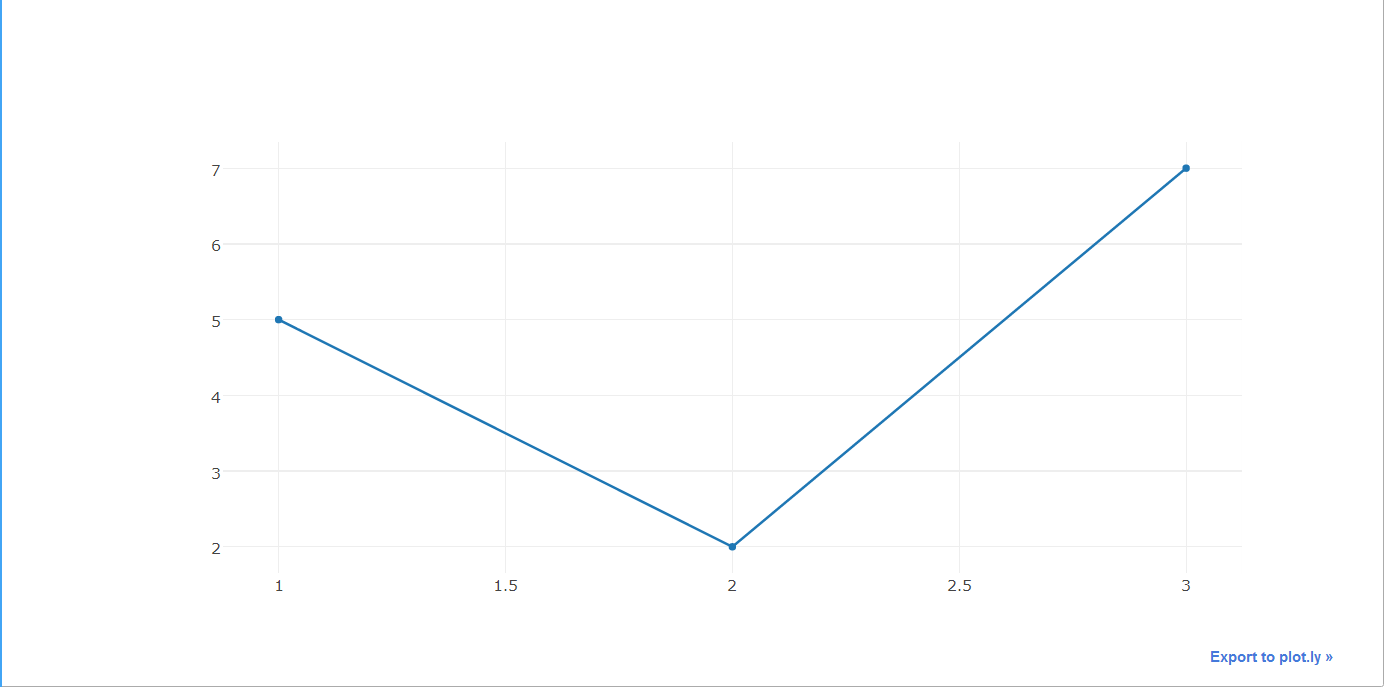2.2 graph对象

plotly中的graph_objs是plotly下的子模块，用于导入plotly中所有图形对象，在导入相应的图形对象之后，便可以根据需要呈现的数据和自定义的图形规格参数来定义一个graph对象，再输入到plotly.offline.iplot()中进行最终的呈现，查询相关帮助手册得到如下结果：

Help on package plotly.graph_objs in plotly:

NAME
plotly.graph_objs

DESCRIPTION
graph_objs
==========

This package imports definitions for all of Plotly's graph objects. For more
information, run help(Obj) on any of the following objects defined here.

The reason for the package graph_objs and the module graph_objs is to provide
a clearer API for users.

PACKAGE CONTENTS
_area
_bar
_box
_candlestick
_carpet
_choropleth
_cone
_contour
_contourcarpet
_deprecations
_figure
_figurewidget
_frame
_heatmap
_heatmapgl
_histogram
_histogram2d
_histogram2dcontour
_layout
_mesh3d
_ohlc
_parcoords
_pie
_pointcloud
_sankey
_scatter
_scatter3d
_scattercarpet
_scattergeo
_scattergl
_scattermapbox
_scatterpolar
_scatterpolargl
_scatterternary
_splom
_surface
_table
_violin
area (package)
bar (package)
box (package)
candlestick (package)
carpet (package)
choropleth (package)
cone (package)
contour (package)
contourcarpet (package)
graph_objs
graph_objs_tools
heatmap (package)
heatmapgl (package)
histogram (package)
histogram2d (package)
histogram2dcontour (package)
layout (package)
mesh3d (package)
ohlc (package)
parcoords (package)
pie (package)
pointcloud (package)
sankey (package)
scatter (package)
scatter3d (package)
scattercarpet (package)
scattergeo (package)
scattergl (package)
scattermapbox (package)
scatterpolar (package)
scatterpolargl (package)
scatterternary (package)
splom (package)
surface (package)
table (package)
violin (package)

DATA
absolute_import = _Feature((2, 5, 0, 'alpha', 1), (3, 0, 0, 'alpha', 0...

FILE
d:\anaconda\lib\site-packages\plotly\graph_objs\__init__.py

2.3 构造traces

在根据绘图需求从graph_objs中导入相应的obj之后，接下来需要做的事情是基于待展示的数据，为指定的obj配置相关参数，这在plotly中称为构造traces(create traces)，下面举两个简单的例子来帮助理解这个部分：

首先，我们来绘制一个较为基本的散点图：

import plotly
import plotly.graph_objs as go
import numpy as np

'''构造1000个服从二维正态分布的模拟数据'''
N = 1000
random_x = np.random.randn(N)
random_y = np.random.randn(N)

'''构造trace，配置相关参数'''
trace = go.Scatter(
x = random_x,
y = random_y,
mode = 'markers'
)

'''将trace保存于列表之中'''
data = [trace]

'''启动绘图'''
plotly.offline.init_notebook_mode()
plotly.offline.iplot(data, filename='basic-scatter')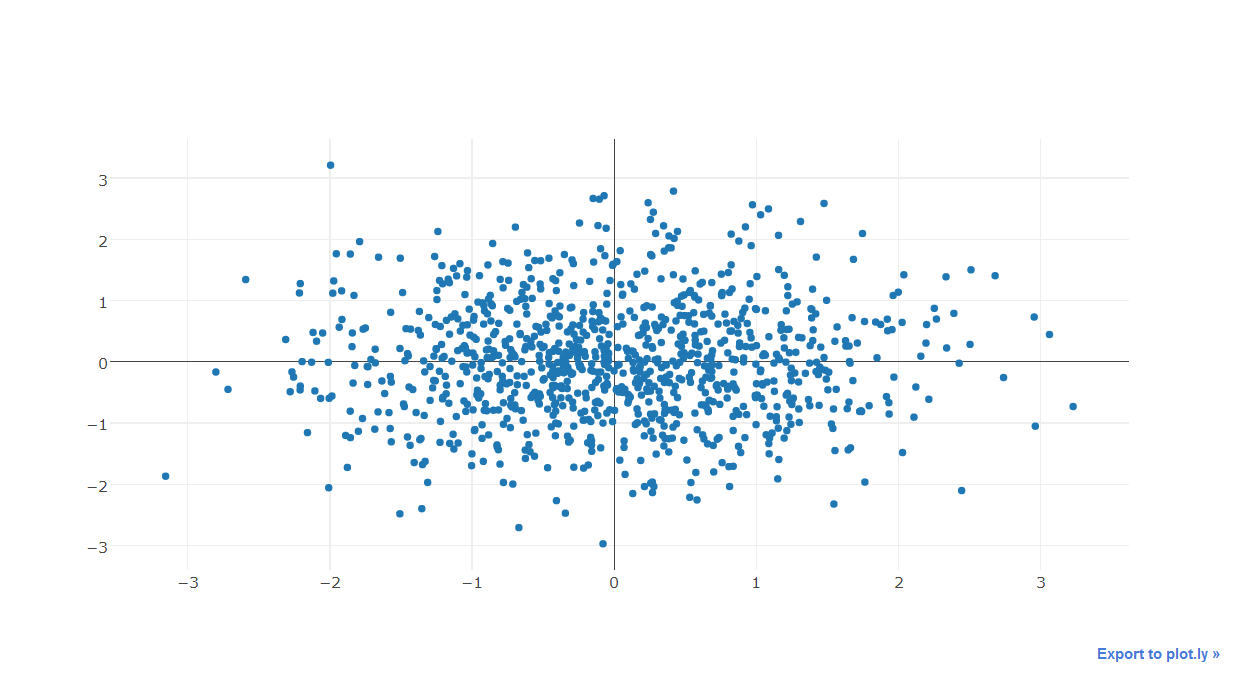从上面的简单实例可以看出trace创建的大致方式，而一张图中可以叠加多个trace，如下面的例子：

import numpy as np
import plotly
import plotly.graph_objs as go

'''创建仿真数据'''
N = 100
random_x = np.linspace(0, 1, N)
random_y0 = np.random.randn(N)+5
random_y1 = np.random.randn(N)
random_y2 = np.random.randn(N)-5

'''构造trace0'''
trace0 = go.Scatter(
x = random_x,
y = random_y0,
mode = 'markers',
name = 'markers'
)

'''构造trace1'''
trace1 = go.Scatter(
x = random_x,
y = random_y1,
mode = 'lines+markers',
name = 'lines+markers'
)

'''构造trace2'''
trace2 = go.Scatter(
x = random_x,
y = random_y2,
mode = 'lines',
name = 'lines'
)

'''将所有trace保存在列表中'''
data = [trace0, trace1, trace2]

'''启动绘图'''
plotly.offline.init_notebook_mode()
plotly.offline.iplot(data, filename='scatter-mode')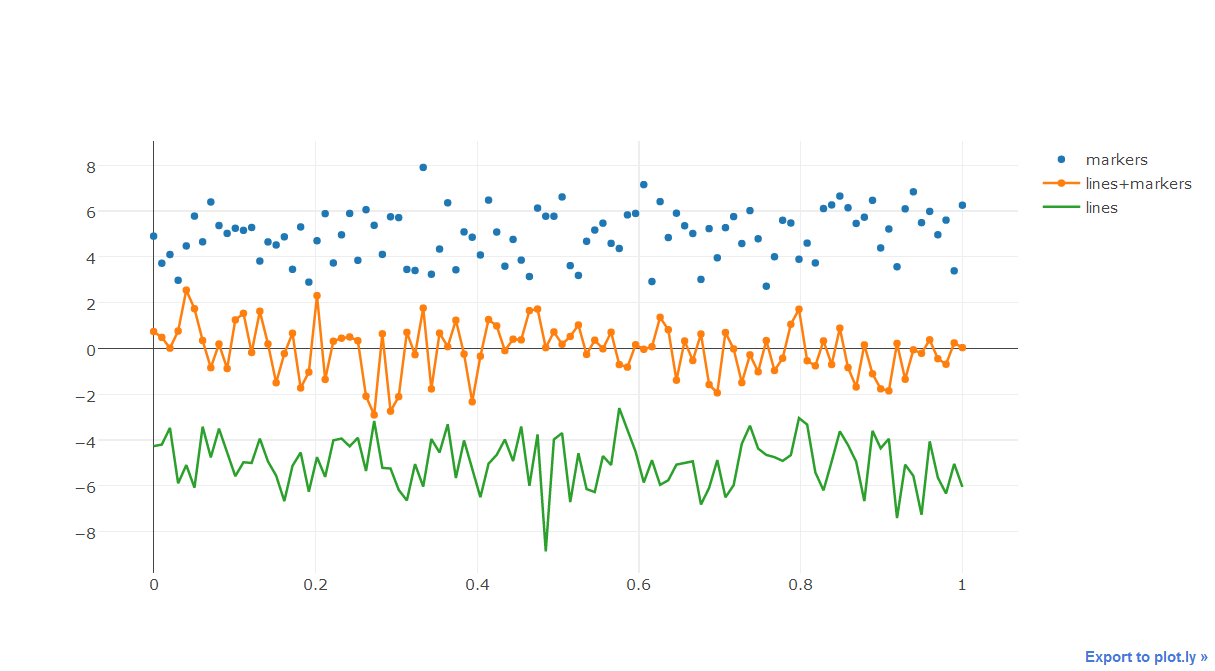2.4 定义Layout

plotly中图像的图层元素与底层的背景、坐标轴等是独立开来的，在我们通过前面介绍的内容，定义好绘制图像需要的对象之后，可以直接绘制，但如果想要在背景图层上有更多自定义化的内容，就需要定义Layout()对象，其主要参数如下，我们根据设置对象的不同分为几个部分并分开举例讲解：

2.4.1 文字

文字是一幅图中十分重要的组成部分，plotly其强大的绘图机制为一幅图中的文字进行了细致的划分，可以非常有针对性地对某一个组件部分的字体进行个性化的设置：

font：字典型，用于控制图像中全局字体的部分，其常用键及功能如下：

family：str型，用于控制字体，默认为'Open Sans'，可选项有'verdana'，'arial'，'sans-serif'等等，具体自行移步官网说明文档

size：int型，用于控制字体大小，默认为12

color：str型，传入十六进制色彩，默认为'#444'

import plotly
import plotly.graph_objs as go
import numpy as np

'''构造1000个服从二维正态分布的模拟数据'''
N = 1000
random_x = np.random.randn(N)
random_y = np.random.randn(N)

'''构造trace，配置相关参数'''
trace = go.Scatter(
x = random_x,
y = random_y,
mode = 'markers'
)

'''将trace保存于列表之中'''
data = [trace]

'''创建layout对象'''
layout = go.Layout(title='测试',
font={
'size':22,
'family':'sans-serif',
'color':'9ed900'#将全局字体颜色设置颜色为葱绿
})

'''将graph部分和layout部分组合成figure对象'''
fig = go.Figure(data=data, layout=layout)

'''启动绘图直接绘制figure对象'''
plotly.offline.init_notebook_mode()
plotly.offline.iplot(fig,filename='basic-scatter')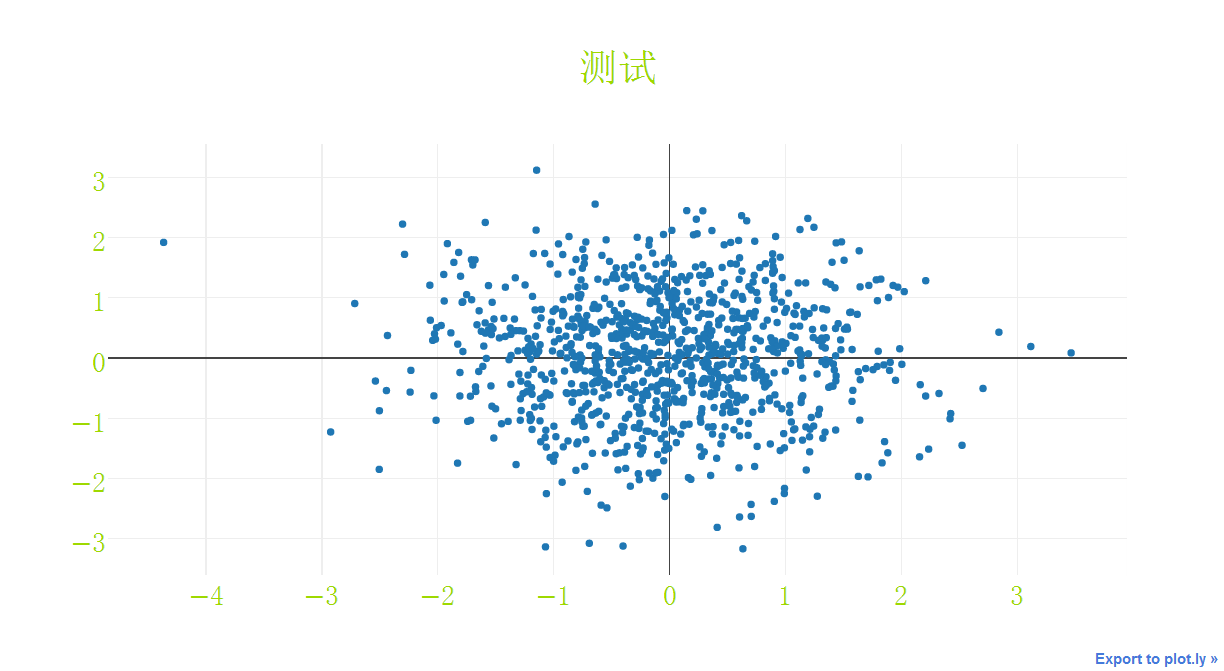title：str型，用于控制图像的主标题

titlefont：字典型，用于独立控制标题字体的部分，其常用键如下：

family：同font中的family，用于单独控制标题字体

size：int型，控制标题的字体大小

color：同font中的color

下面是一个简单的例子：

import plotly
import plotly.graph_objs as go
import numpy as np

'''构造1000个服从二维正态分布的模拟数据'''
N = 1000
random_x = np.random.randn(N)
random_y = np.random.randn(N)

'''构造trace，配置相关参数'''
trace = go.Scatter(
x = random_x,
y = random_y,
mode = 'markers'
)

'''将trace保存于列表之中'''
data = [trace]

'''创建layout对象'''
layout = go.Layout(title='测试',
titlefont={
'size':20,
'color':'9ed900'#将标题字体颜色设置颜色为葱绿
})

'''将graph部分和layout部分组合成figure对象'''
fig = go.Figure(data=data, layout=layout)

'''启动绘图直接绘制figure对象'''
plotly.offline.init_notebook_mode()
plotly.offline.iplot(fig,filename='basic-scatter')2.4.2 坐标轴

xaxis或yaxis：字典型，控制横坐标的各属性，其主要键如下：

color：str型，传入十六进制色彩，控制横坐标上所有元素的基础颜色（在未单独指定颜色之前，这些元素均采用此处color指定的颜色）

title：str型，设置横坐标轴上的标题

titlefont：字典型，同之前所有同名参数

type：str型，用于控制横坐标轴类型，'-'表示根据输入数据自适应调整，'linear'表示线性坐标轴，'log'表示对数坐标轴，'date'表示日期型坐标轴，'category'表示分类型坐标轴，默认为'-'

autorange：bool型或'reversed'，控制是否根据横坐标对应的数据自动调整坐标轴范围，默认为True

range：list型，控制横坐标轴的区间范围，自行设置无默认项，取决于横坐标轴的数据类型，格式均为[左端点,右端点]

tickmode：str型，设置坐标轴刻度的格式，'auto'表示自动根据输入的数据来决定，'linear'表示线性的数值型，'array'表示由自定义的数组来表示（用数组来自定义刻度标签时必须选择此项）

tickvals：list、numpy array或pandas中的series，作为坐标轴刻度标签的替代（tickmode此时必须被设置为'array'）

ticks：str型，控制刻度标签的书写位置，'outside'表示在外侧显示，'inside'表示在内侧显示，''表示不显示

ticklen：int型，设置刻度标签的像素长度

tickwidth：int型，设置刻度标签的像素宽度

tickcolor：str型，传入十六进制色彩，用于控制刻度标签的颜色

tickfont：字典型，同前面所有字典型字体控制参数，用于对刻度标签进行单独控制

tickangle：int型，设置刻度标签的旋转角度

showline：bool型，控制是否绘制出该坐标轴上的直线部分

linecolor：str型，十六进制色彩，控制坐标轴线条的色彩

linewidth：int型，设置坐标轴直线部分的像素宽度

showgrid：bool型，控制是否绘制网格线

gridcolor：str型，十六进制色彩，控制网格线的颜色

gridwidth：int型，控制网格线的像素宽度

zeroline：bool型，控制是否在0值处绘制0刻度线

side：str型，控制x(y)轴放置于作图区域的位置，'top'、'bottom'控制横轴放置于顶部亦或是底部；'left'、'right'控制纵轴放置于左侧亦或是右侧

下面是几个简单的示例。

1.对横纵坐标轴标题字体进行修改

import plotly
import plotly.graph_objs as go
import numpy as np

'''构造1000个服从二维正态分布的模拟数据'''
N = 1000
random_x = np.random.randn(N)
random_y = np.random.randn(N)

'''构造trace，配置相关参数'''
trace = go.Scatter(
x = random_x,
y = random_y,
mode = 'markers'
)

'''将trace保存于列表之中'''
data = [trace]

'''创建layout对象，对横纵坐标轴的标题进行一定的设置'''
layout = go.Layout(xaxis={
'title':'这是横坐标轴',
'titlefont':{
'size':30
}
},yaxis={
'title':'这是纵坐标轴',
'titlefont':{
'size':40
}
})

'''将graph部分和layout部分组合成figure对象'''
fig = go.Figure(data=data, layout=layout)

'''启动绘图直接绘制figure对象'''
plotly.offline.init_notebook_mode()
plotly.offline.iplot(fig,filename='basic-scatter')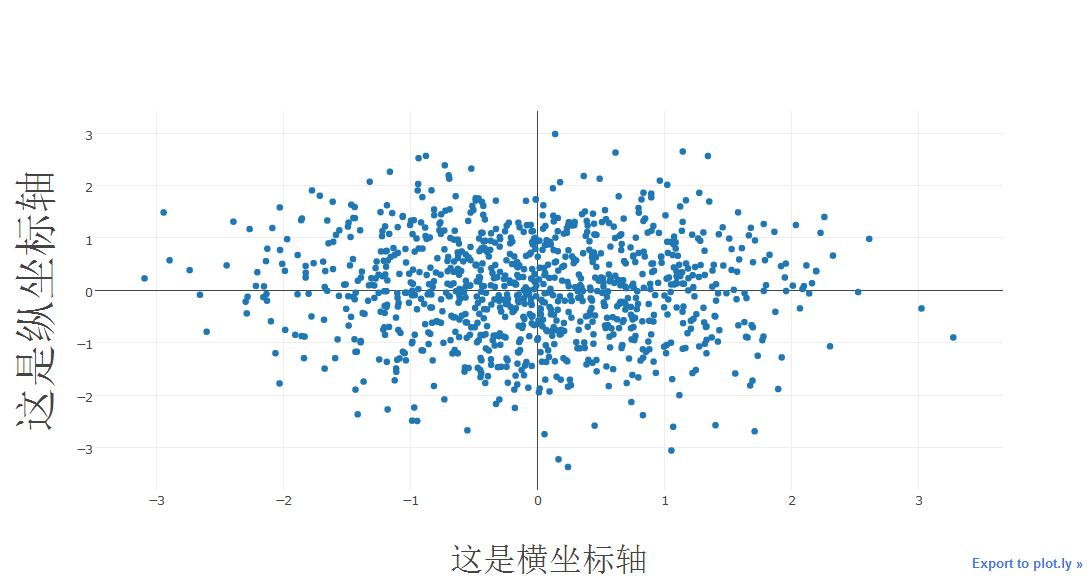2.对横纵方向的坐标轴线条及网格进行设置

import plotly
import plotly.graph_objs as go
import numpy as np

'''构造1000个服从二维正态分布的模拟数据'''
N = 1000
random_x = np.random.randn(N)
random_y = np.random.randn(N)

'''构造trace，配置相关参数'''
trace = go.Scatter(
x = random_x,
y = random_y,
mode = 'markers'
)

'''将trace保存于列表之中'''
data = [trace]

'''创建layout对象，对横纵坐标轴的线条及网格颜色进行一定的设置'''
layout = go.Layout(xaxis={
'showline':False,
'showgrid':True,
'zeroline':False,
'showgrid':True,
'gridcolor':'7fecad'
},yaxis={
'showline':False,
'showgrid':True,
'gridcolor':'#3d3b4f',
'zeroline':False
})

'''将graph部分和layout部分组合成figure对象'''
fig = go.Figure(data=data, layout=layout)

'''启动绘图直接绘制figure对象'''
plotly.offline.init_notebook_mode()
plotly.offline.iplot(fig,filename='basic-scatter')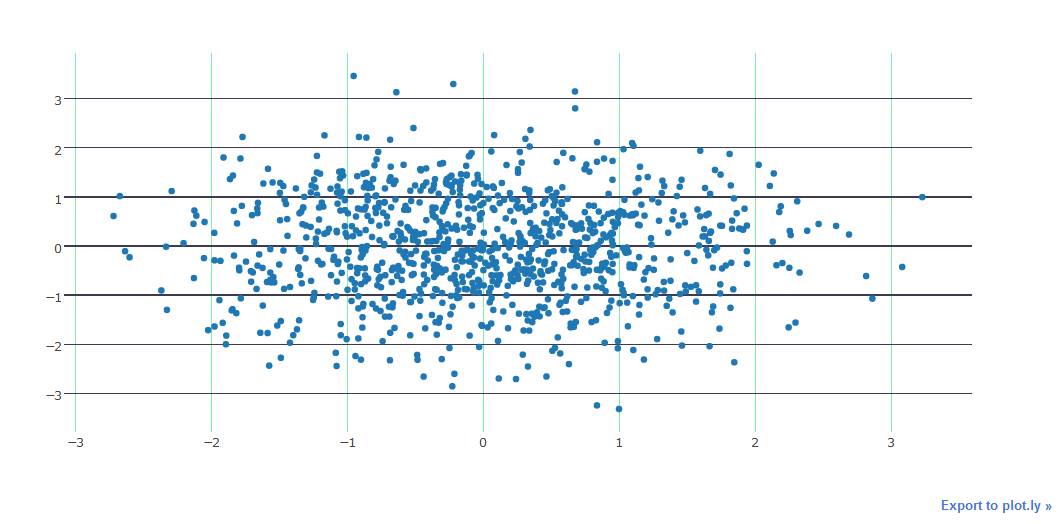2.4.3 图例

showlegend：bool型，控制是否绘制图例

legend：字典型，用于控制用图例相关的所有属性的设置，主要键如下：

bgcolor：str型，十六进制设置图例背景颜色

bordercolor：设置图例边框的颜色

borderwidth：int型，设置图例边框的颜色

font：字典型，设置图例文字部分的字体，同前面所有font设置规则

orientation：str型，设置图例各元素的堆叠方向，'v'表示竖直，'h'表示水平堆叠

x：数值型，-2到3之间，用于设置图例在水平方向上的位置，默认为1.02

xanchor：str型，用于直接设置图例水平位置的固定位置，有'left'、'center'、'right'和'auto'几个可选项

y：数值型，-2到3之间，用于设置图例在竖直方向上的位置，默认为1

yanchor：str型，用于直接设置图例竖直方向上的固定位置，有'top'、'middle'、'bottom'和'auto'几个选项

import numpy as np
import plotly
import plotly.graph_objs as go

'''创建仿真数据'''
N = 100
random_x = np.linspace(0, 1, N)
random_y0 = np.random.randn(N)+5
random_y1 = np.random.randn(N)
random_y2 = np.random.randn(N)-5

'''构造trace0'''
trace0 = go.Scatter(
x = random_x,
y = random_y0,
mode = 'markers',
name = 'markers'
)

'''构造trace1'''
trace1 = go.Scatter(
x = random_x,
y = random_y1,
mode = 'lines+markers',
name = 'lines+markers'
)

'''构造trace2'''
trace2 = go.Scatter(
x = random_x,
y = random_y2,
mode = 'lines',
name = 'lines'
)

'''将所有trace保存在列表中'''
data = [trace0, trace1, trace2]

'''构造layout对象，对图例位置进行一定的设置'''
layout = go.Layout(legend={
'x':0.5,
'y':0.5
})

'''构造figure对象'''
fig = go.Figure(data=data,layout=layout)

'''启动绘图'''
plotly.offline.init_notebook_mode()
plotly.offline.iplot(fig, filename='scatter-mode')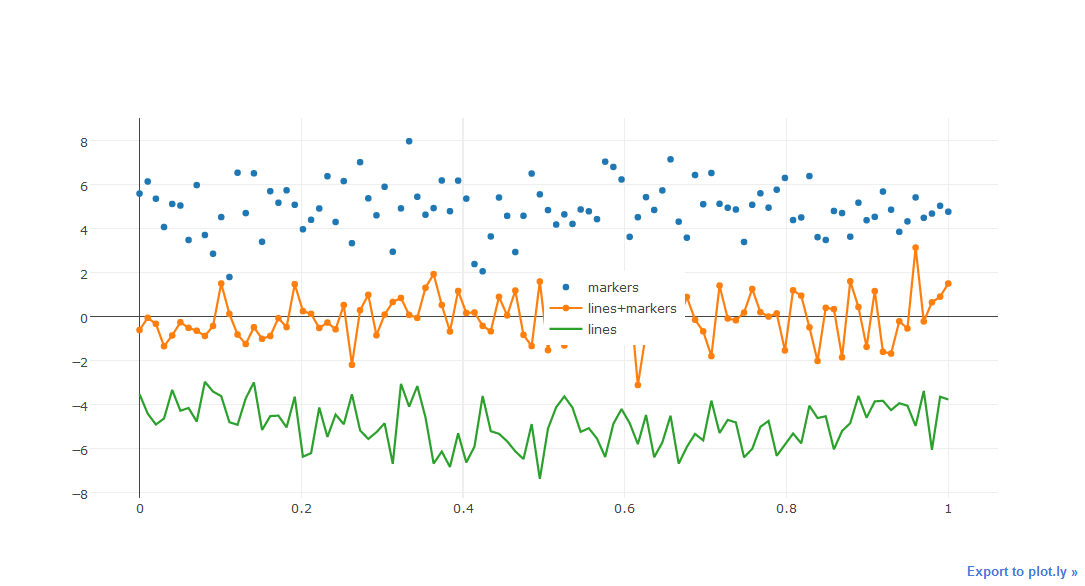2.4.4 其它杂项

width：int型，控制图像的像素宽度，默认为700

height：int型，控制图像的像素高度，默认为450

margin：字典型输入，控制图像边界的宽度，其主要键如下：

l：int型，控制图像距离左边界的留白区域像素宽度，默认为80

r：int型，控制图像距离右边界的留白区域像素宽度，默认为80

t：int型，控制图像距离上边界的留白区域像素宽度，默认为100

b：int型，控制图像距离下边界的留白区域像素宽度，默认为80

pad：int型，控制坐标轴与图像区域的像素距离，默认为0

paper_bgcolor：str型，传入十六进制色彩，控制图床的颜色

plot_bgcolor：str型，传入十六进制色彩，控制绘图区域的颜色

hidesources：bool型，控制是否在图像的右下角标记来源link

hovermode：str型或False，用于设置悬停交互的方式，有'x'、'y'、'closest'和False这几个可选项，False表示无悬停交互方式

hoverlabel：字典型输入，用于控制悬停时出现的信息框的各属性，主要键如下：

bgcolor：str型，传入十六进制色彩，控制信息框的背景色

bordercolor：str型，传入十六进制色彩，控制信息框边框的颜色

font：字典型，控制信息框中字体的各属性，其主要键如下：

family：同之前，控制字体

size：int型，控制字体大小，默认13

color：str型，传入十六进制色彩，控制字体颜色

namelength：int型，控制对信息框中显示的对应trace的名字的长度限制，建议设置为-1，即全部显示，默认为15，即对于长于15的trace只显示前15个字符

grid：字典型，控制一页多图（subplots）时的规划多个图的网格的属性，其常用键如下：

rows：int型，控制网格中的行数（放置笛卡尔坐标系类型的子图），也可以设置多于实际绘图需求的行数以达到留白的目的

roworder：str型，设置子图按行，是从下往上叠加还是从上往下叠加，对应'top to bottom'和'bottom to top'，默认为'top to bottm'，注意，只可以设置行的叠加顺序，列方向上的叠加顺序始终为从左往右

columns：int型，同rows，控制网格的列数

pattern：str型，用于控制一页多图中子图之间坐标轴的共享情况，'coupled'表示每一列共享同一个x轴，每一行共享一个y轴，'independent'表示每个子图xy轴独立（这在进行量纲相差较大的子图的绘制尤为有用）

xgap：float型，0.0-1.0之间，用于控制子图之间的水平空白区域宽度占一个子图宽度的百分比

ygap：同xgap，控制竖直方向上子图之间的宽度

domain：字典型，设置一页多图时，子图占据的区域距离上下左右边界的宽度情况，其主要键如下：

x：list型，格式为[x1,x2]，x1控制子图区域左端与图床左端的距离，x2控制子图区域右端与图床左端的距离，x1、x2都代表百分比，在0.0-1.0之间取值

y：同x，控制子图区域上下端分别与图床上端的距离百分比

以上就是plotly的绘图基础部分，如有笔误，望指出。

*这里提供一个十六进制颜色对照表以辅助调色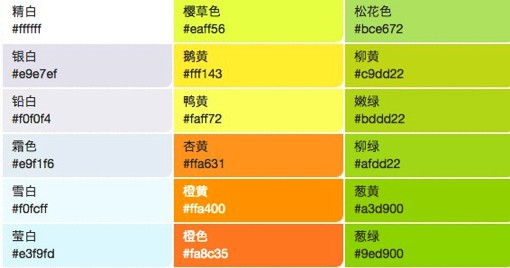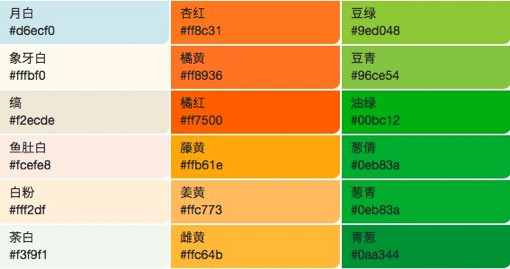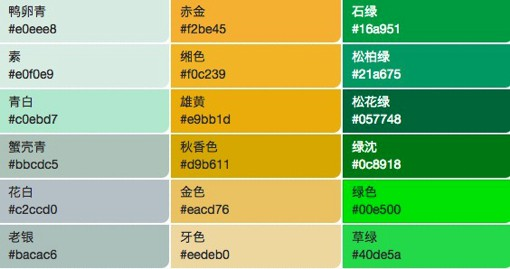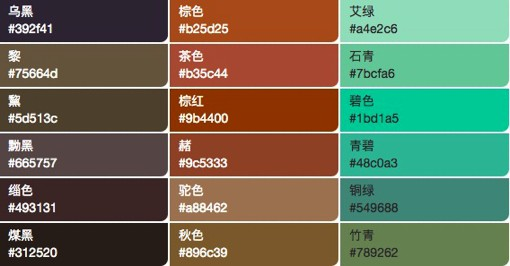posted @ 2018-07-12 22:21  费弗里  阅读(16809)  评论(2编辑  收藏  举报